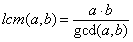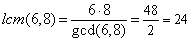Math Calculators, Lessons and Formulas

It is time to solve your math problem

mathportal.org

# GCD and LCM calculator

This calculator will find Greatest Common Divisor (GCD) and Least Common Multiple (LCM) of two or more numbers. Step-by-step explanation is provided.

GCD and LCM Calculator
0 1 2 3 4 5 6 7 8 9 - , del
 Greatest Common Divisor (GCD) Least Common Multiple (LCM)

GCD stands for Greatest Common Divisor. GCD is largest number that divides the given numbers.

### GCD Example

Find the GCD of 45 and 54.

Step 1: Find the divisiors of given numbers:

The divisiors of 45 are : 1, 3, 5, 9, 15, 45

The divisiors of 54 are : 1, 2, 3, 6, 9, 18, 27, 54

Step 2: Find the greatest number that these two lists share in common. In this example the GCD is 9.

LCM stands for Lowest Common Multiple. LCM is the smallest number that is a multiple of both a and b.

### LCM Example

Find the LCM of 6 and 8.

The multiples of 6 are : 6, 12, 18, 24, 30, . . .

The multiples of 8 are : 8, 16, 24, 32, 40, . . .

So, the Lowest Common Multiple is 24.

Also, we can calculate the LCM using the following formula:Now we can find the LCM of 6 and 8 as:Quick Calculator Search